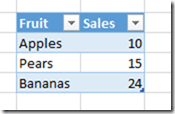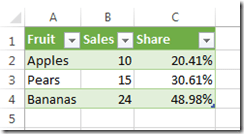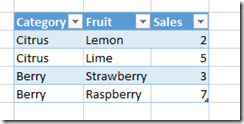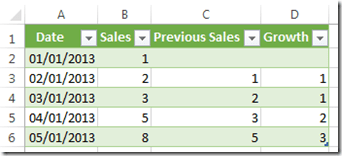# Implementing Common Calculations In Power Query

When I first saw Data Explorer (as Power Query used to be called), I immediately started thinking of it as a self-service ETL tool; indeed, it’s how Microsoft itself is positioning it. However I suspect that a lot of Power Query users might also want to use it for building reports too: it could be that they find DAX too difficult, and if all they need is a simple table-based report they might not want to bother with using Power Pivot at all.

If that happens then it’s going to be important to be able implement common business calculations in Power Query… hence this blog post. I’ll also admit that I wanted to know how easy it is to write these calculations – because if it isn’t easy, or if it isn’t easier than DAX or the equivalent Excel formulas, then no-one will bother doing it. So let’s look at some examples.

Simple Percentage Share

Take the following table in Excel as a starting point:Now let’s imagine that we want to find the percentage that each fruit makes up of the grand total (ie 10+15+24=49). The way to calculate the percentage is quite easy: you need to find the grand total, then divide the sales for each fruit by the grand total. Finding the grand total requires some code though – you might think you could do it easily in the UI using the Group By functionality, but that does not allow you to sum up all the values in a column and not group by anything! (I hope this gets fixed soon). Luckily the code is quite easy: you use Table.Column() to convert the Sales column into a List, then use List.Sum() to sum up all the values in that List. Finally, you insert a new custom column to the original table and calculate the percentage. Here’s the code:

`let`

`    Source = Excel.CurrentWorkbook(){[Name="Table1"]}[Content],`

`    Sales = Table.Column(Source, "Sales"),`

`    GrandTotal = List.Sum(Sales),`

`    TableWithShare = Table.AddColumn(Source, "Share", each [Sales]/GrandTotal)`

`in`

`    TableWithShare`It would also be nice if I could get the table that Power Query outputs to remember the number formatting I apply to it after it refreshes… again, I hope this gets fixed.

Nested Share

A more complex example of a Share calculation might be where we need a share of a subtotal. Consider the following table:In this case, let’s say we need to show each Fruit’s sales as a percentage of the total sales of its Category. This time we can use the Group By functionality to get a table containing the subtotals very easily. Next, we can join our subtotal table with the original table using Table.Join() so we have the subtotals on each row. Finally, we can do the share calculation and remove the subtotal column again. Here’s the code:

`let`

`    Source = Excel.CurrentWorkbook(){[Name="Table4"]}[Content],`

`    Subtotals = Table.Group(Source, {"Category"}, {{"Category Sales", `

`        each List.Sum([Sales]), type number}}),`

`    JoinTables = Table.Join(Source, "Category", Subtotals, "Category"),`

`    InsertedCustom = Table.AddColumn(JoinTables, "Share", each [Sales]/[Category Sales]),`

`    RemovedColumns = Table.RemoveColumns(InsertedCustom,{"Category Sales"})`

`in`

`    RemovedColumns`Previous Period Growth

Now consider a table with a date column (Americans: the dates are in dd/mm/yyyy format) and a sales column:Let’s say we want to find the absolute change in Sales from the previous date to the current date on each row. Here we need to copy the table, shift the dates forward one day (by adding a value of type Duration onto the date) and then join the original table onto this new table using Table.Join() so we can see the current day sales and the previous day sales in two columns next to each other; this will then mean we can subtract one from the other to find the growth. Here’s the code:

`let`

`    Source = Excel.CurrentWorkbook(){[Name="Table7"]}[Content],`

`    ChangedType = Table.TransformColumnTypes(Source,{{"Date", type date}}),`

`    InsertedCustom = Table.AddColumn(ChangedType, "Next Date", each [Date]+#duration(1,0,0,0)),`

`    RemovedColumns = Table.RemoveColumns(InsertedCustom,{"Date"}),`

`    RenamedColumns = Table.RenameColumns(RemovedColumns,{{"Sales", "Previous Sales"}}),`

`    JoinTables = Table.Join(ChangedType, "Date", RenamedColumns, "Next Date",JoinKind.LeftOuter),`

`    RemovedColumns1 = Table.RemoveColumns(JoinTables,{"Next Date"}),`

`    SortedRows = Table.Sort(RemovedColumns1,{{"Date", Order.Ascending}}),`

`    Growth = Table.AddColumn(SortedRows, "Growth", each [Sales]-[Previous Sales])`

`in`

`    Growth`Year-to-Date Sales

For the final example, take the same starting table and before and now let’s calculate a year-to-date running sum. In this case, I decided to use a function (see here for some background on this, and here for a more complex example of a function) to do the calculation. Here’s the code of the function, called YTD(), that I created:

`let`

`    YTD = (SalesTable, EndDate) =>`

`    let`

`        FilteredTable=Table.SelectRows(SalesTable, `

`            each [Date]<=EndDate and Date.Year([Date])=Date.Year(EndDate) ),`

`        SalesColumn = Table.Column(FilteredTable, "Sales"),`

`        YTDSales = List.Sum(SalesColumn)`

`    in`

`        YTDSales`

`in`

`    YTD`

It takes a table structured in the same way as the source table (ie it has to have a [Date] column and a [Sales] column), filters the data using Table.SelectRows() so only rows with a date less than or equal to the EndDate parameter, but in the same year as it, are returned, and then sums up the values in the [Sales] column of the filtered table.

It’s then very easy to use this function in a new calculated column in a query:

`let`

`    Source = Excel.CurrentWorkbook(){[Name="Table7"]}[Content],`

`    ChangedType = Table.TransformColumnTypes(Source,{{"Date", type date}}),`

`    InsertedCustom = Table.AddColumn(ChangedType, "YTD Sales", each YTD(ChangedType, [Date]))`

`in`

`    InsertedCustom`

And voila, we have a column containing the year-to-date sum:Conclusion

All of these examples here required me to write M code, and to be honest this is not something an ordinary user will ever be able to do. Furthermore, I suspect that end users will always prefer to write their calculations as Excel formulas, although more complex calculations such as nested shares might prove beyond them. However, I believe that a good data steward will certainly be able to write M code, and the last example above makes me think that if a data steward can write a function that performs a calculation and shares it with the end users, the end users should be able to use it in their own queries quite easily. I don’t think the code I’ve written here is any worse or better than the DAX equivalent but the ability to share functions could tip the balance in favour of Power Query for some simple reporting scenarios.

### 28 responses

1.David Hager (@dhExcel) says:

Thanks for sharing, Chris. Your latest example made me start to wonder where the Query is stored, and if an Excel file can be identified programmatically as having a Query.

•Chris Webb says:

The query is stored inside the Excel workbook; I’m not sure how exactly though.

•David Hager (@dhExcel) says:

I see how now. The provider for PQ queries is:

Microsoft.Mashup.OleDb.1

So, I can open files programmatically and loop through the connections to see if that provider exists.

2.waltika says:

Reblogged this on waltika.

3.Steven Peters says:

Chris

I too have been comparing powerquery (M) to powerpivot (DAX) as a tool for analysis. There is one area already that I believe powerquery has the advantage and that is when you need to generate a list of members based on the results of an initial query – obvious example is the common task of creating a histogram. Dynamically converting measures into members via dax is not easy. I have also been comparing sql with m as a language for analysts – definitely some plus points for powerquery here.

4.Mike Honey says:

Thanks Chris – some great stuff there, so thanks for sharing. I’m enjoying Power Query also – I find the coding/debugging experience is already much better than Excel Formulas or DAX. It’s certainly 100 times easier than SSIS or similar ETL tools.

I got the idea from your Year-to-Date Sales function to write a function that returns a Running Count / Index within a group – I just pass an extra function parameter for the current group, then add that test to the Table.SelectRows criteria. Final change was to change List.Sum to List.Count.

5.sam says:

@Chris

Here is one more way of doing Running Totals.

Assuming you have a 2 Column Table of Month (Jan,Feb…) and Sales (10,30…) and the Table is called S
The below M Code generates a Cumulative Sales Column called cSales

let
Source = Excel.CurrentWorkbook(){[Name=”S”]}[Content],
mTotal = Table.AddColumn(mTbl, “Total”, each Table.Range(mTbl,0,[Index]+1)),
cTotal = Table.AggregateTableColumn(mTotal, “Total”, {{“Sales”, List.Sum, “cSales”}}),
rColumn = Table.RemoveColumns(cTotal,{“Index”})
in
rColumn

I have no Idea how the performance compares with your method…but I was able to build this via the UI – so for someone not very proficient with M yet this looks easier.

•Chris Webb says:

Thanks!

6. Pingback: Sum in Power Query

7.moncherwatson says:

Hi Chris, I need to do a nested share (just like the one in your example above) but I must end up with the cumulative % for each category. How would you do that?

8.Jimmy says:

Hi Chris and team,

I’ve got an fairly complex variation of your example above, but i’m running into some roadblocks and hoping you could provide some guidance.

Basically, I’m generating a dynamic list of employee hours – these are categorized by hours per day for multiple employees. My goal is to automatically “code” the hours as overtime or regular time, based on the US regulations for those hours (for instance, 40 hours per week would be considered regular time, anything over that are considered overtime).

The difficulty I’m running into is creating a “running” tally of the hours by employee by the Date.WeekOfYear.

It’s laid out sort of like these three columns:
John Doe – 3/13/15 – 9 hours
Bob Employee – 3/14/15 – 12 hours
John Doe – 3/14/15 – 12 hours
Bob Employee – 3/16/15 – 12 hours

I’m trying to dynamically calculate a fourth column which would show the running total for the week:
John Doe – 3/13/15 – 9 hours – 9 hours
Bob Employee – 3/14/15 – 12 hours – 12 hours
John Doe – 3/14/15 – 12 hours – 21 hours
Bob Employee – 3/16/15 – 12 hours – 24 hours

Then reset each week and start the calculation again. I’ve used a variation of your function above, and it outputs a running tally, but it’s not a) separated by week and b) separated by individual employee.

Any recommendations?

JDH

•Chris Webb says:

I can think of a way to do this, but as always the real challenge is to come up with an *easy* way to do this. Maybe you could try something like this:
1) Create a Power Query function that adds a running total column to a table that only contains data for a single employee for a single week
2) Use Table.Partition() to split your current table up into a list of tables that each contain data for just one employee and one week
3) Apply the function created in (1) to every table in the list in step (2)
4) Combine the list of tables back into a single table

•Jimmy says:

Thanks, Chris. Conceptually, that’s what I was thinking, but would I drop that into the function itself or into the query using the function?

The other idea rattling around my head was if there was a way to dynamically group the table itself by more than one column. In other words, within the function, i’m creating a running tally of hours by filtering the table by the date, but would there be a way to further filter that or group that by individual names (other than the table.partition recommendation)? The function would filter (Table.SelectRows) by name first, then filter by hours based on date (using Table.SelectRows again).

I’m not sure if I’m smart enough to know if PQ could accomplish this within one function or not. Perhaps building two separate functions and link them together in the main query? Any ideas?

•Jimmy says:

Ok – so after much head bashing, I think I got the specifics worked out so it’s a ton easier than I expected. Using a derivation of your function, I simply added a third variable for the name, then made that part of the FilteredTable line using Table.SelectRows like this:

FilteredTable=Table.SelectRows(HoursTable, each ([Date]<=EndDate and Date.WeekOfYear([Date])=Date.WeekOfYear(EndDate)) and [Name]=FullName),

That ended up slicing everything out exactly how I needed it…it creates a column of the running weekly tally of hours by employee. Phew…apparently some unnecessary pain overall. Thanks for the guidance.

Jimmy

•Jimmy says:

So after my solution, I ran into more issues…basically, using the current function that i have implemented for this solution:
let
WTD = (HoursTable, EndDate,FullName,HourIndex) =>
let
FilteredTable=Table.SelectRows(HoursTable, each ([Index]<=HourIndex and Date.WeekOfYear([WeekModDate])=Date.WeekOfYear(EndDate)) and [Full Name]=FullName),
HoursColumn = Table.Column(FilteredTable, "Payable Time"),
WTDHours = List.Sum(HoursColumn)
in
WTDHours
in
WTD

I'm having massive loading delays…for instance, right now i have a little over 800 rows of data, but it's taking a solid 10-12 minutes to load the query. This will only get worse as data is continued to populate each day…

I'm guessing the delay is caused by the function cycling through the entire list of data for each row. Do you have any recommendations on how I could possibly streamline this function to hone in on the relevant data and speed up the load time? I'm at a big sticking point.

•Chris Webb says:

Maybe a better approach would be to think in SQL, expand all the rows, join the table to itself with the appropriate join condition then do a group by?

•ImkeF says:

Hi Jimmy,
wondering why you want to keep the daily granularity. Wouldn’t it just be so easy to:

1) Group by week & employee and aggregate sum on hours
2) Add the weekly regular time in a column and subtract

Imke

•Jimmy says:

lmke,

This is funny timing because I actually did a similar setup with Chris Webb on this situation this morning right before i saw your comment. Basically, joined the table with itself, grouped on Week and Name, then ran a function to create a running tally that I had mentioned above…the join was key, as it reduced my load time substantially and actually made the query usable again.

I need both the weekly running total and a daily running total because of the way the OT laws are set up by state in the US – some states require OT to be based on daily amounts, others on the weekly amounts.

•ImkeF says:

Jimmy,
that sounds interesting: What was your join condition?
How long does your query run now on 800 rows?

•Jimmy says:

Before these tweaks to it, it was running about 1700 rows in >2minutes. After the tweaks, it was running it in under 10 seconds, so fairly dramatic improvements.

Right now, I’ve built a much more complex model incorporating this (and a whole lot more) and it’s running on close to 4000 rows in about a minute…if it was JUST the joins referenced above, it would probably run in about 10 seconds, but the other code (including merges, appends and some API calls) slow it down a bit. I’ve found that buffering the tables works pretty well to shorten that as much as possible, but still just fairly complex all around.

9.ImkeF says:

Thanks for coming back on this.
This self-merge on your table structure just seemed so clever. Still not exactly knowing which conditions you use, but with this approach, you wouldn’t even need the function to be invoked after (also quicker):

let
Source = Excel.CurrentWorkbook(){[Name=”Table”]}[Content],
Merge = Table.NestedJoin(Source,{“Name”, “Week”, “Year”},Source,{“Name”, “Week”, “Year”},”NewColumn”),
Expand = Table.ExpandTableColumn(Merge, “NewColumn”, {“Name”, “Date”, “Hours”, “Week”, “Year”}, {“Name.1”, “Date.1”, “Hours.1”, “Week.1”, “Year.1”}),
AddCol = Table.AddColumn(Expand, “Filter”, each if [Date.1] <= [Date] then 1 else 0),
Filter = Table.SelectRows(AddCol, each ([Filter] = 1)),
Group = Table.Group(Filter, {"Date", "Name", "Week"}, {{"Cum", each List.Sum([Hours]), type number}})
in
Group

•Jimmy says:

hmmm…I see where you’re going on this, but there may be a little more to my model that needs to be extrapolated upon. The curve ball here is based on the possibility that an employee would submit multiple hours (aka rows) for a single day, so your grouping won’t work in that situation. Basically, on the download from the server, I’m sorting by date, then adding an index column which creates a sequential structure that “catches” the multi-hour entries in one day. That’s why I ended up invoking the function, which (when combined with the self-merge) allows me to sort by date, week number, employee and index.

•ImkeF says:

Oh I see – there you go 🙂
THX again

10.Erik A says:

Hi Chris,

Thanks for this article! No other place on the web has information on how to calculate a share of something within something else in power query. It helped me a lot. It took forever though for me to figure out how to implement it with my columns and table names. But it works now!

I also had more than the one column you have in the example to join on, I had 3 joins. But found that I could use Table.NestedJoin.

Cheers!

11.Fractal76 says:

The running total solutions above appear to work only for very small dataset sizes, before running into O(N^2) bottleneck (because doing cumulative sum of all items before current items, requires N*N/2 scans through the full list)

I found this solution using List.Generate to work much better (several orders faster)

Table2 has a “Value” column that is being accumulated.

let
tbl = Excel.CurrentWorkbook(){[Name=”Table2″]}[Content],
vals = List.Buffer(Table.Column(tbl, “Value”)),
totalCt = List.Count(vals),
out = List.Generate( () => [idx=0, s = vals{0}], each [idx] < totalCt, each [idx=[idx]+1, s = vals{idx} + [s]], each [s])
in
out

•Chris Webb says:

Yes, that makes a lot of sense. Thanks for sharing!

12.LENZY PETTY says:

Can you do year comparisons in Power Query. For example,
OEM – Date – Volume
GM – 1/1/2016 100
GM – 1/2017 150

Can I add a column that will show
OEM – Date – Volume – New Column Diff
GM – 1/1/2016 100
GM – 1/2017 150 – 50

This site uses Akismet to reduce spam. Learn how your comment data is processed.